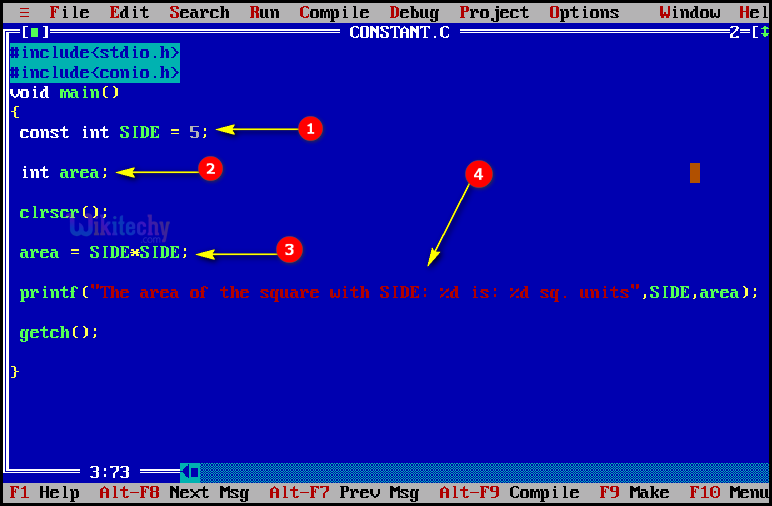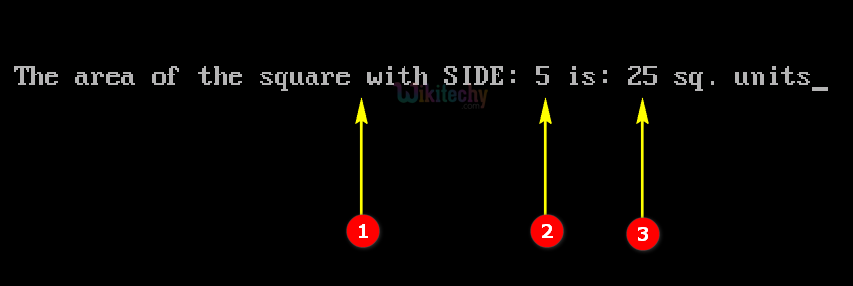# C - ConstantLearn C - C tutorial - C constant - C examples - C programs

## C Constant

• A constant is a value that never changes.
• Constant in C means the content whose value does not change at the time of execution of a program.
• Constants are also called literals.
• It is considered as the best exercise to define constants using only upper-case names.## Types of Constant :

• Integer Constant
• Real constant
• Character constant
• Backslash character constant

## Integer constant :

• An "integer constant" is a decimal (base 10), octal (base 8), or hexadecimal (base 16) number that represents an integral value.
• Integer constants are used to represent integer values that cannot be changed.

## Real constant :

• Constants in C are fixed value that does not change during the execution of a program
• A real constant is combination of a whole number followed by a decimal point and the fractional part.

## Character constant :

• A character constant is a single alphabet , a single digit or a single special symbol enclosed within a pair of single quotes.

## Backslash character constant :

• There are some characters which have special meaning in C language.
• They should be preceded by backslash symbol to make use of special function of them.
• C supports some special backslash character constants that are used in output functions.
 \\ \ character \' ' character \" " character \? ? character \n New line Formation \r Carriage return \f Form feed \a Alert or bell \b Backspace \v Vertical tab \t Horizontal tab \ooo Octal number of one to 3 digits \xhh . . . Hexadecimal number of one or more digits

## C Code - Explanation :1. In C programming, const int SIDE =5 is a constant integer data type that specifies the side value as 5.
2. In C programming, int is specified as integer data type with its variable name as area.
3. In this example, here we are calculating area , where area=SIDE*SIDE .
4. In this example, “The area of the square with SIDE” is represented as a printf statement , where %d is an integer datatype assigning the value of 5 with its unit as sq. unit

## Sample Output - Programming Examples1. Here in this output “The area of the square with SIDE” is represented as a printf statement which is being called in the console window.
2. Here in this output 5 specifies the integer variable.
3. Here in this output 25 specifies the area square of integer value 5 .